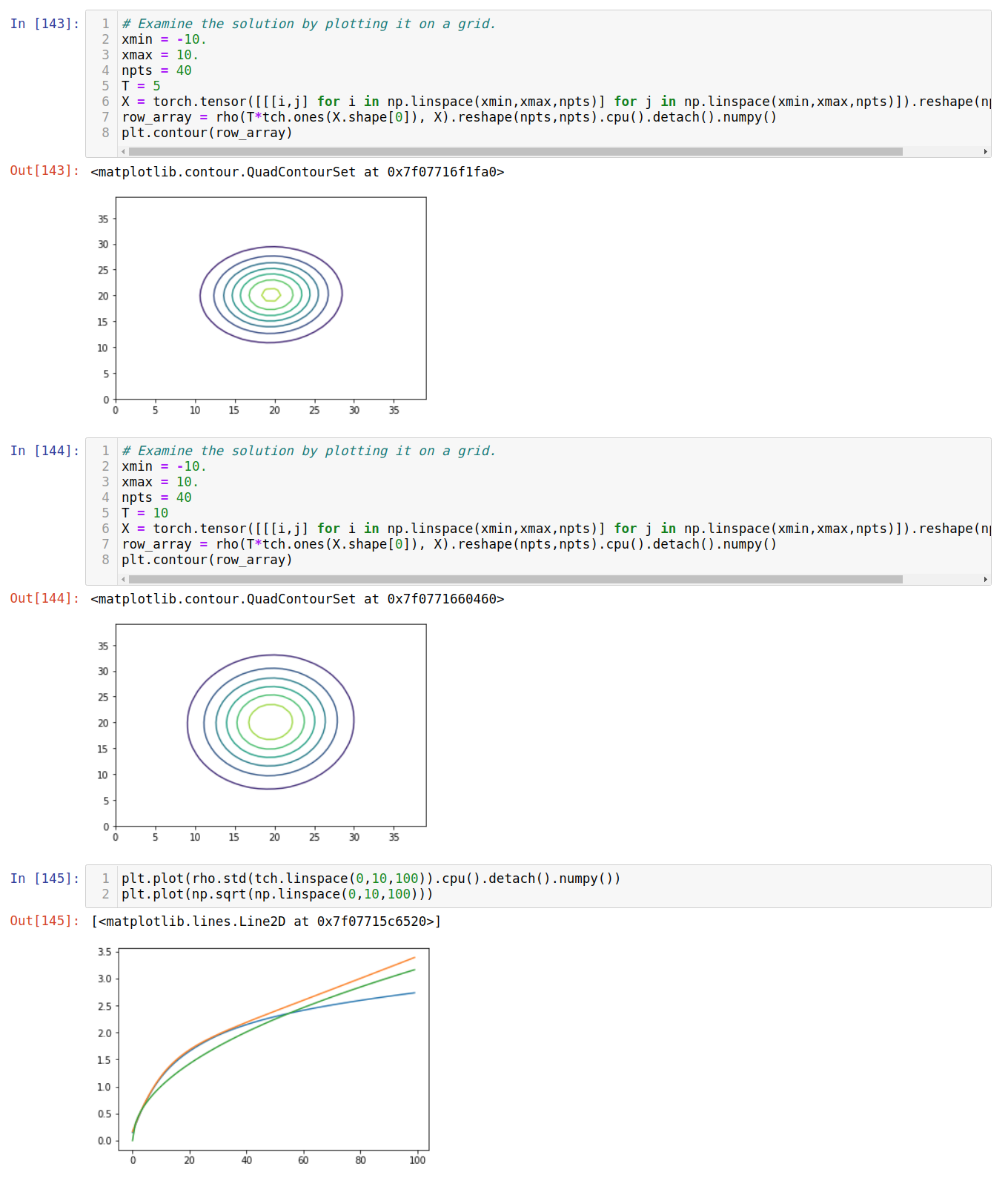Solving multi-dimensional partial differential equations (PDE’s) is something I’ve spent most of my adult life doing. Most of them are somewhat similar to the heat equation:

$\nabla^2 u = \frac{du}{dt}$

where u is some function of possibly many variables. Liouville’s equation, the Schrodinger equation, The Fokker-Plank equation etc. etc. are all equations of this form. Usually one spends as much time trying to derive useful properties from u, as one spends solving u.

Many properties of $$u(\vec{x},t)$$ of interest are actually derivatives or integrals, perhaps even of the solution with respect to parameters of the equation or solver or vice versa. To this end, it’s extraordinarily useful to be able to solve this equation in such a way that u is differentiable with respect to these parameters and vice-versa.

For example, to calibrate the parameters $$\mu (X_{t},t), \sigma (X_{t},t)$$ of a multidimensional stochastic differential equation: $$dX_{t}=\mu (X_{t},t)\,dt+\sigma (X_{t},t)\,dW_{t}$$ one would often like the conditional density $$P(X_t | X_{t-1})$$ which is available as a solution to a Fokker-Plank equation:

${\frac {\partial P(\mathbf {x} ,t)}{\partial t}}=-\sum _{i=1}^{N}{\frac {\partial }{\partial x_{i}}}\left[\mu _{i}(\mathbf {x} ,t)P(\mathbf {x} ,t)\right]+\sum _{i=1}^{N}\sum _{j=1}^{N}{\frac {\partial ^{2}}{\partial x_{i}\,\partial x_{j}}}\left[D_{ij}(\mathbf {x} ,t)P(\mathbf {x} ,t)\right]$

with respect to a delta function initial condition. The applications to finance should now be more obvious, this is how one calibrates Heston or more sophisticated models. Usually one would need hundreds of lines of code to optimize $$\mu (X_{t},t), \sigma (X_{t},t)$$, it’s actually the subject of innumerable theses, and several luminaries of econometrics are famous for simply applying Galerkin methods to optimize these parameters.

In all my years of solving PDEs… I have never really come across a simpler way to solve them than what I’m about to show (<100 lines!). This also results in a solution which is differentiable. It’s all quick, and very general. The only limitation is that derivatives above 2 would be impractical, but besides that very high dimensions are solvable.

# A solution of the heat equation in pytorch.

The basic idea here is to use the incredible approximation properties of neural networks as a “more better” Galerkin method. Simply sample a very flexible differentiable function and force it to obey conditions in batches.

• step 1: build a flexible neural ansatze.
• step 2: implement the PDE.
• step 3: minimize error of the PDE under the ansatze.
• step 4: visualize.

I will show two ansatze, a simple feed-forward network, and a feed-forward Gaussian Mixture model. If you don’t know much about Gaussian mixtures, you can just look at the simplicity of the neural density. Both simply model a function $$\rho(t,x)$$. The advantage of the Gaussian Mixture is that normalization is automatically enforced, and it could easily be used for importance sampling. Ideally one could use normalizing flows or any other sophisticated method to generate a flexible distribution.

Next we define a module which checks that the heat equation is satisfied on a random batch of points. These could be chosen more cleverly by importance sampling etc. but this is sufficient to show the correct behavior.

Finally we merely optimize these losses:

Now note that the correct solution of the heat equation in two dimensions results in the standard deviations of a growing Gaussian blob, growing with $$\sim \sqrt(t)$$ Here’s what images look like from our differentiable solution:Not too shabby for such little work and time and so much generality.